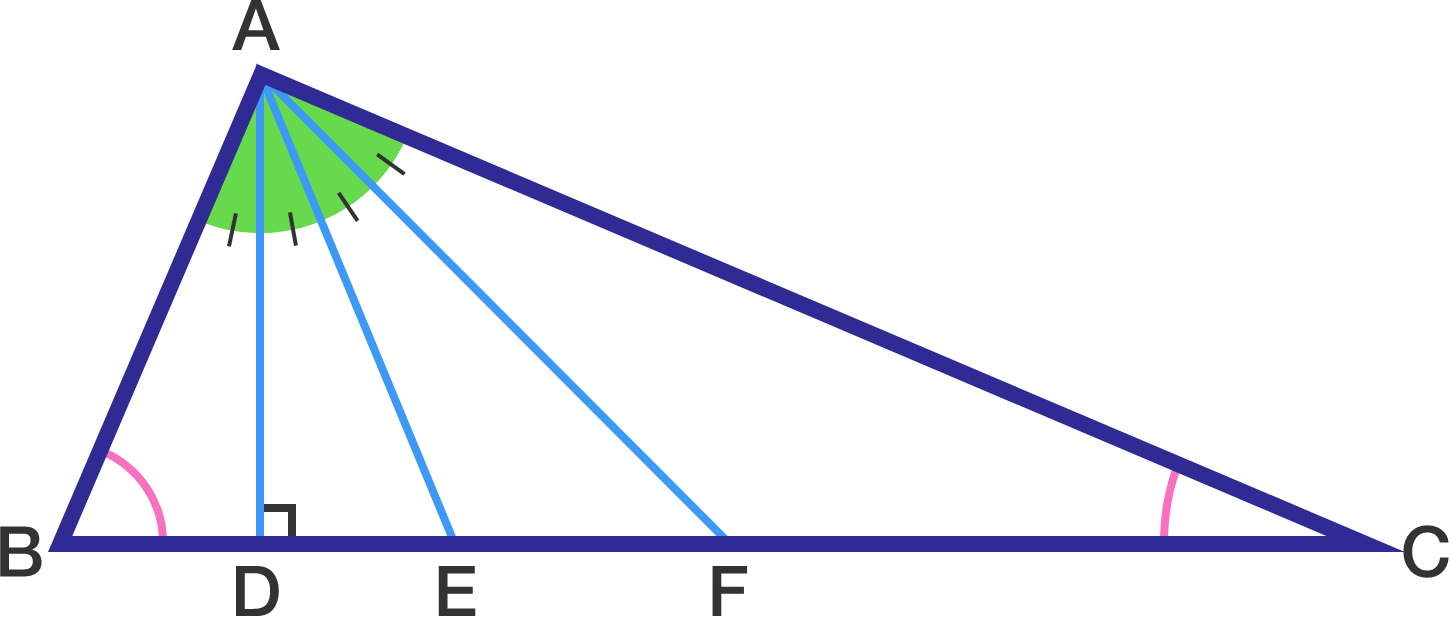# Do you like evaluating the angles of a Triangle?

Geometry Level 3In a $\Delta ABC$, the altitude, the angle bisector and the median from $A$ divides the $\angle A$ into four equal angles. Find the value of $\bigg| \angle B-\angle C \bigg|$ in degrees.

×

Problem Loading...

Note Loading...

Set Loading...